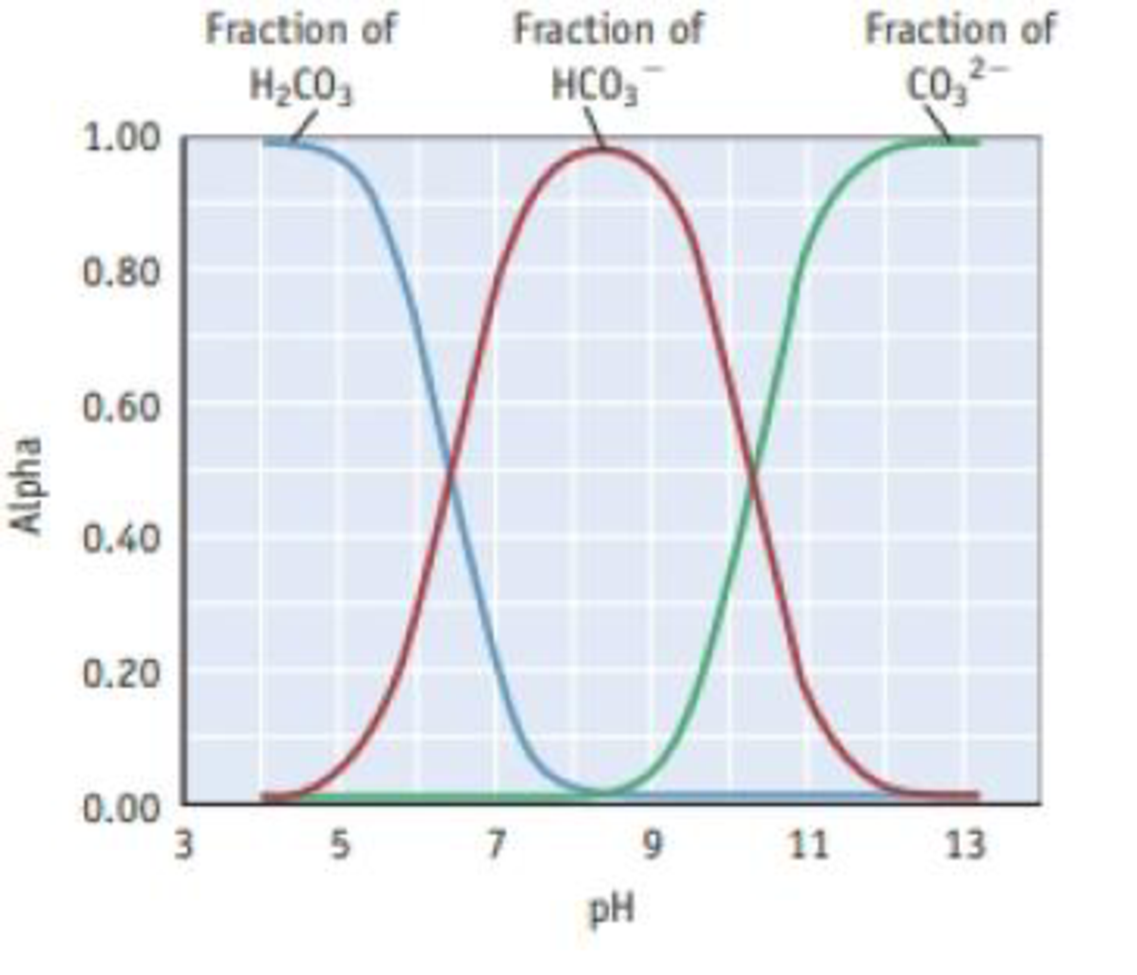Chapter 20, Problem 48GQ

Chapter
Section
Textbook Problem

Refer to the figure below and Figure 20.25, which show the fraction of species in solution [alpha (α)] as a function of pH. The following questions are in regard to the equilibria involved in an aqueous solution of H2CO3 (dissolved CO2) in fresh water at 25 °C. (a) At what pH does |H2CO3(aq)| = |HCO3−|? (b) At what pH does [HCO3−| = |CO32−|? (c) What is the predominant species in solution when the solution has a pH of 8? (d) What species are in solution when the solution has a pH of 7?(a)

Interpretation Introduction

Interpretation: The pH at which [H2CO3]=[HCO3-] should be determined using the given graph.

Concept introduction:

Ocean acidification: The increase in concentration of carbon-dioxide in the atmosphere leads to ocean acidification. The amount is increasing day by day and this increase will lead to higher concentrations of dissolved CO2 and as a result the amount of carbonic acid also will be higher.

The concentration of hydronium ion is a key factor for many biochemical reactions. So variation may affect the organisms in the oceans.

The relationship between ocean pH and atmospheric CO2 is that they are inversely proportional. The hydrogen ion concentration will be increased as the concentration of dissolved CO2 increases and thus pH will be decreased.

Explanation

If the concentration of dissolved CO2 is increased, then the pH will be decreased as a result of the increase in concentration of hydrogen ion.

The graph showing the fraction of species in the solution as a function of pH is given below.

From the graph we can identify the pH at which the

(b)

Interpretation Introduction

Interpretation: The pH at which the [HCO3-]=[CO32-] should be determined using the given graph.

Concept introduction:

Ocean acidification: The increase in concentration of carbon-dioxide in the atmosphere leads to ocean acidification. The amount is increasing day by day and this increase will lead to higher concentrations of dissolved CO2 and as a result the amount of carbonic acid also will be higher.

The concentration of hydronium ion is a key factor for many biochemical reactions. So variation may affect the organisms in the oceans.

The relationship between ocean pH and atmospheric CO2 is that they are inversely proportional. The hydrogen ion concentration will be increased as the concentration of dissolved CO2 increases and thus pH will be decreased.

(c)

Interpretation Introduction

Interpretation: The predominant species in the solution when the pH is 8 should be determined.

Concept introduction:

Ocean acidification: The increase in concentration of carbon-dioxide in the atmosphere leads to ocean acidification. The amount is increasing day by day and this increase will lead to higher concentrations of dissolved CO2 and as a result the amount of carbonic acid also will be higher.

The concentration of hydronium ion is a key factor for many biochemical reactions. So variation may affect the organisms in the oceans.

The relationship between ocean pH and atmospheric CO2 is that they are inversely proportional. The hydrogen ion concentration will be increased as the concentration of dissolved CO2 increases and thus pH will be decreased.

(d)

Interpretation Introduction

Interpretation: The predominant species in the solution when the pH7 should be determined.

Concept introduction:

Ocean acidification: The increase in concentration of carbon-dioxide in the atmosphere leads to ocean acidification. The amount is increasing day by day and this increase will lead to higher concentrations of dissolved CO2 and as a result the amount of carbonic acid also will be higher.

The concentration of hydronium ion is a key factor for many biochemical reactions. So variation may affect the organisms in the oceans.

The relationship between ocean pH and atmospheric CO2 is that they are inversely proportional. The hydrogen ion concentration will be increased as the concentration of dissolved CO2 increases and thus pH will be decreased.

Still sussing out bartleby?

Check out a sample textbook solution.

See a sample solution

The Solution to Your Study Problems

Bartleby provides explanations to thousands of textbook problems written by our experts, many with advanced degrees!

Get Started